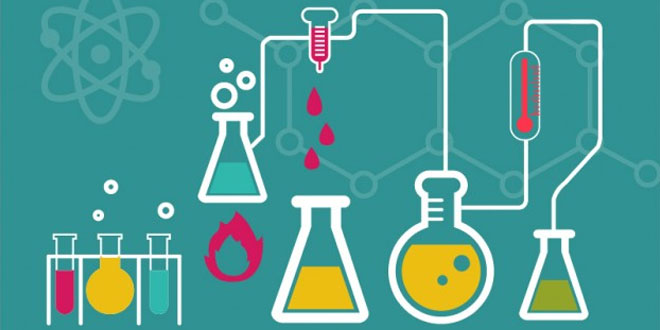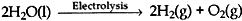Wednesday , May 18 2022# Chemical Reaction & Equations: 10 Science

#### Question: Explain the following terms with one example each. 1. Corrosion 2. Rancidity

1. Corrosion is the process in which metals are eaten up gradually by the action of air, moisture or a chemical on their surface. Corrosion is caused mainly by the oxidation of metals by the oxygen of air.

Example:
Rusting of iron is the most common form of corrosion. When an iron object is left in damp air for a considerable period of time, it gets covered with a red-brown flaky substance called “rust”. This is called rusting of iron.
2. The condition produced by aerial oxidation of fat and oil in food which is marked by an unpleasant smell and taste is called rancidity.Example: Rancidity can be retarded by keeping food in a refrigerator.The refrigerator has a low temperature inside it. When the food is kept in a refrigerator, the oxidation of fat and oil in it is slowed down due to low temperature. So, the development of rancidity due to oxidation is retarded.

#### Question: Why should a magnesium ribbon be cleaned before burning in air?

Answer: Magnesium ribbon should be cleaned with sandpaper before burning in air in order to remove white layer of magnesium oxide (MgO) from its surface which hinders the burning of magnesium.

#### Question: Write the balanced equation for the following chemical reactions: (1) Hydrogen + Chloride → Hydrogen chloride (2) Barium chloride + Aluminium sulphate → Barium sulphate + Aluminium chloride (3) Sodium + Water → Sodium hydroxide + Hydrogen

1. To balance a chemical equation:
Hydrogen + Chlorine → Hydrogen chloride
Step I: Writing the skeletal chemical equation:
H2 + Cl2 HCl
Step II: Balancing the equation:
H2 + Cl2 → 2HCl
2. To balance a chemical equation:
Barium chloride + Aluminium sulphate Barium sulphate + Aluminium chloride
Step I: Writing the skeletal chemical equation:
BaCl2 + Al2 (SO4)3 → BaSO4 + AlCl3
Step II: Balancing the equation:
3BaCl2 + Al2(SO4)3 → 3BaSO4 + 2AlCl3
3. To balanced a chemical equation:
Sodium + water Sodium hydroxide + Hydrogen
Step I: Writing the skeletal chemical equation:
Na + H2O → NaOH + H2
Step II:
Balancing the equation:
2Na + 2H2O → 2HaOH + H2

#### (2) Sodium hydroxide solution (in water) react with hydrochloric acid solution (in water) to produce sodium chloride solution and water.

1. To balanced a chemical equation:
Barium chloride + Sodium sulphate → Barium sulphate + Sodium chloride
(solution)                       (solution)                  (Insoluble solid)               (solution)
Step I: Writing the skeletal chemical equation:
BaCl2 (aq) + Na2SO4 (aq) → BaSO4(s) + NaCl (aq)
Step II: Balancing the equation:
BaCl2 (aq) + Na2SO4 (aq) → BaSO4(s)↓+ 2NaCl (aq)
2. To balance a chemical equation:
Sodium hydroxide + Hydrochloric acid → Sodium chloride + Water

Step I: Writing the skeletal chemical equation:
NaOH(aq) + HCl(aq) → NaCl(aq) + H2O(l)
Step II:
Balancing the equation:
NaOH(aq) + HCl(aq) → NaCl(aq) + H2O(l)

#### (2) Write reaction of the substance ‘X’ named in (a) above with water.

1. For whitewashing a solution quicklime is used. The substance ‘X’ is quicklime its formula is CaO [Calcium oxide].
2. When quicklime reacts with water, the following reaction takes place:
CaO (s) + H2O(l) → Ca(OH)2(aq)

#### Question: Why is the amount of gas collected in one of the test tubes in Activity 1.7 double of the amount collected in the other? Name this gas.

Answer: During electrolysis, water decomposes to form hydrogen and oxygen gases.In the above reaction, the balanced chemical equation shows that on electrolysis, the water decomposes to from hydrogen and oxygen in the ration of 2:1 by volume.

#### Question: Why does the colour of copper sulphate solution change when an iron nail is dipped into it?

Answer: In this reaction, iron displaces copper from copper sulphate solution. The deep blue colour of copper sulphate solution fades due to the formation of light green solution of iron sulphate. A red-brown coating of copper metal is formed on the surface of the iron metal. This displacement reaction occurs because iron is more reactive than copper.